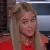Crownnote is where music fans share their favorite songs.

Chart

# My 2015 Year-End Countdown

1. Number
171
2. Number
172
3. Number
173
4. Number
174
5. Number
175
6. Number
176
7. Number
177
8. Number
178
9. Number
179
10. Number
180
11. Number
181
12. Number
182
13. Number
183
14. Number
184
15. Number
185
16. Number
186
17. Number
187
18. Number
188
19. Number
189
20. Number
190
21. Number
191
22. Number
192
23. Number
193
24. Number
194
25. Number
195
26. Number
196
27. Number
197
28. Number
198
29. Number
199
30. Number
200

A chart byjafetsigfinns
Date
Songs
30
No points